### Home > A2C > Chapter 11 > Lesson 11.1.2 > Problem11-28

11-28.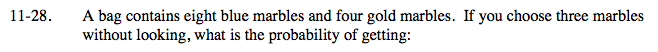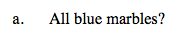$\frac{_8C_3 \cdot _4C_0}{_{12}C_3 }=\frac{14}{55}$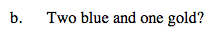$\frac{_8C_2 \cdot _4C_1}{_{12}C_3 }=\frac{28}{55}$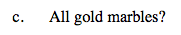See parts (a) and (b).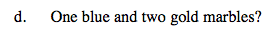See parts (a) and (b).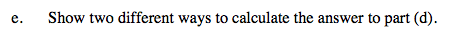How can you solve part (d) using combinations? How can you solve part (d) using the answers to parts (a) through (c)?